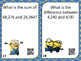# Addition & Subtraction: Computation & Estimation Task Cards with QR CodesSubject
Resource Type
File Type
PDF (1 MB|18 pages)
Standards
\$2.50
• Product Description
• Standards
Make math centers easy with these self-checking QR code task cards. This set of 28 task cards includes addition and subtraction problems. Concepts included in these task cards are:
-Addition and subtraction of up to 6-digit numbers
-Estimation problems
-Single & Multi-Step word problems involving addition and subtraction

These cards are great to use in math centers or while teaching a small group. They would make a great addition to any classroom learning about addition & subtraction of up to 6-digit numbers!

These task cards cover the following standards:
**Common Core Standards: MATH.CONTENT.4.NBT.B.4; 4.OA.A.2
**Virginia Standards of Learning: SOL 4.4

Answer sheet with key is also included!

**I strive for accuracy, so if you notice an error of any kind, please do not hesitate to contact me. My goal is to make high-quality products!
Multiply or divide to solve word problems involving multiplicative comparison, e.g., by using drawings and equations with a symbol for the unknown number to represent the problem, distinguishing multiplicative comparison from additive comparison.
Fluently add and subtract multi-digit whole numbers using the standard algorithm.
Total Pages
18 pages